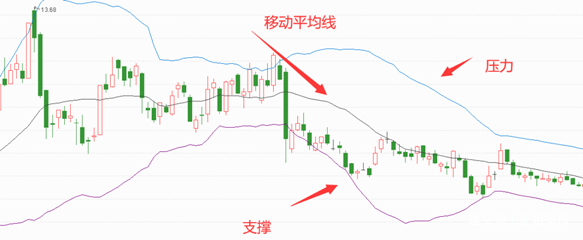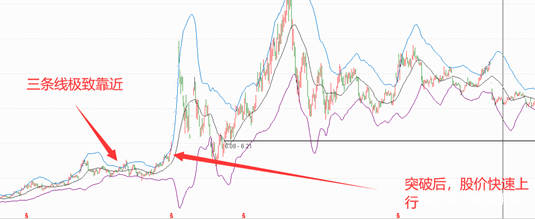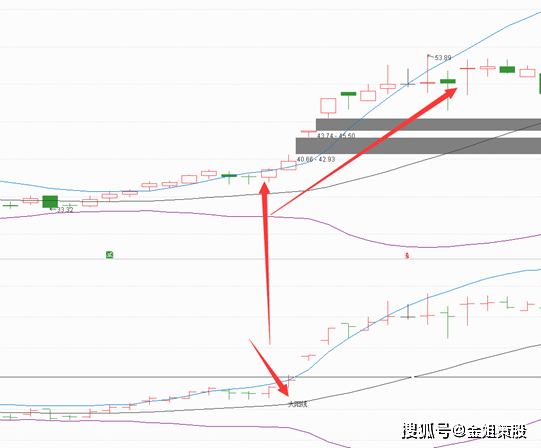# 布林线指标又叫BOLL指标

2021-10-15  搜狐号  金姐策股BOLL:MA(CLOSE,20);

UB:BOLL+2*STD(CLOSE,20);

LB:BOLL-2*STD(CLOSE,20);

A1:=UB/LB<1.25;

A3:=COUNT(A1,20)=20;

A4:=C>MA(C,10) AND C>MA(C,20)AND C>MA(C,30) AND C>MA(C,60) AND C>MA(C,120) AND C>MA(C,250);

A5:=REF(A3,1) AND A4 AND UB/LB>1.25;

DRAWTEXT(A5,BOLL,'大阳线');▍相关推荐